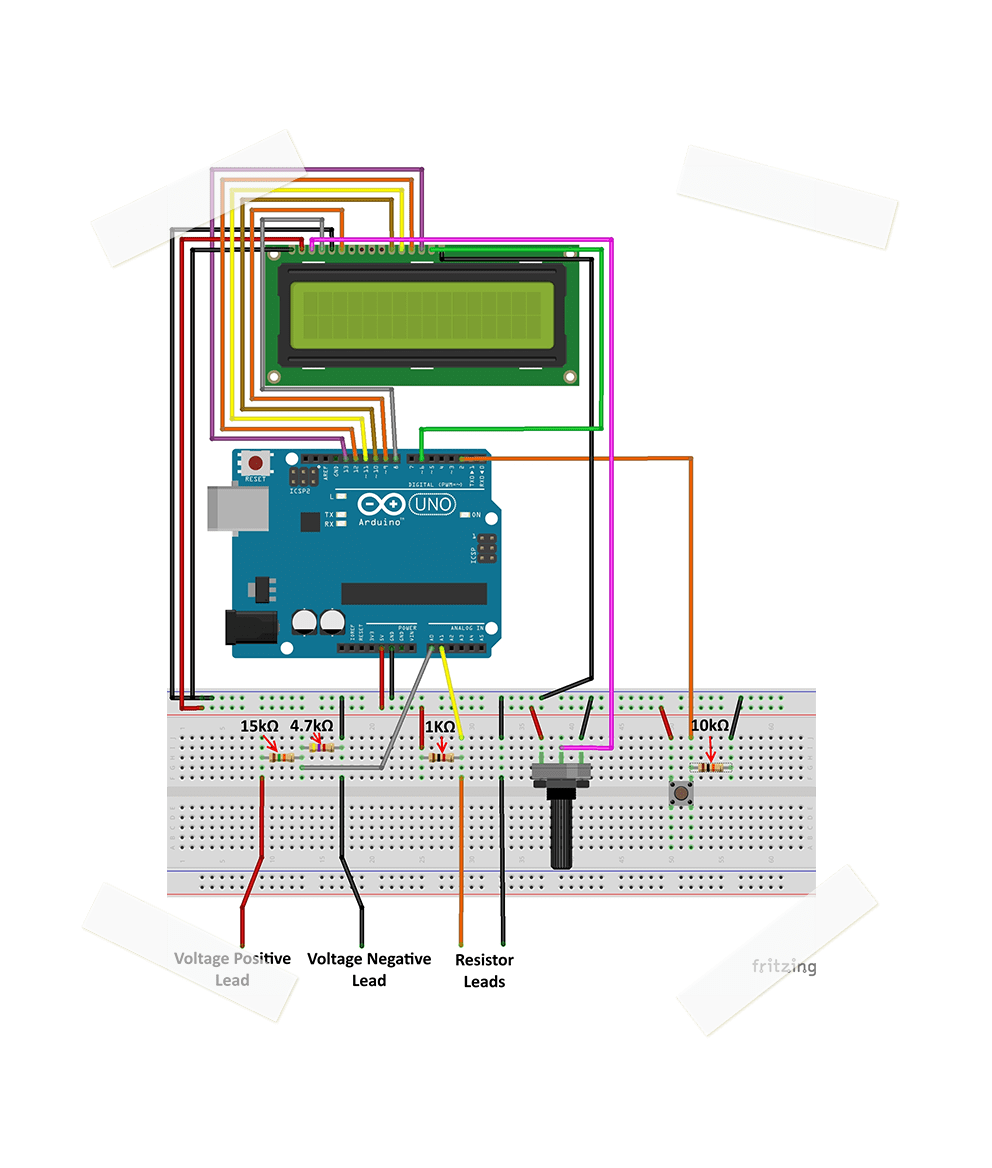# MULTIMETER# EXERCISE SOLUTIONS:

Challenge

Part A:

1.) 20 Volts
2.) 40 Volts
3.) 10 Volts

Part B:

1.) 0.1 Amps
2.) 0.5 Amps
3.) 5 Amps

Question 1

The measurements are most likely not exact. This mainly comes from the fact that the resistors are not perfectly accurate. For example, if the 15kΩ resistor is actually 14.5kΩ, the reading will be slightly higher than expected.

Question 2

While playing with the potentiometer, you will realize that the middle pin is variable while the outer two pins stay fixed at 10kΩ. When measuring between the left and the center pin, the resistance is close to zero when the potentiometer is turned all the way to the left, and about 10kΩ when all the way to the right. When connected to the center and right pins, this is the opposite.

Question 3

When the outer two pins are connected to 5V and GND, the voltage on the middle pin will sweep from approximately 0 to 5 volts. This is because the potentiometer forms a voltage divider, with the ability to adjust the voltage output. The potentiometer's three pins can be seen as two resistors connected together at the middle pin. Because the outer pins always add up to 10kΩ, the denominator in the voltage divider equation always adds up to 10kΩ. This allows the numerator to sweep from 0Ω to 10kΩ depending on the angle of the potentiometer input, and therefore change the output voltage.

Bonus

Download the code above (the 'I Give Up' file) for the solution to this question.